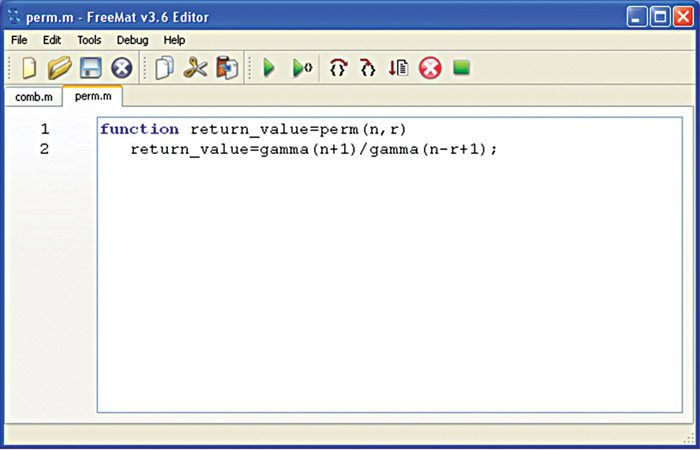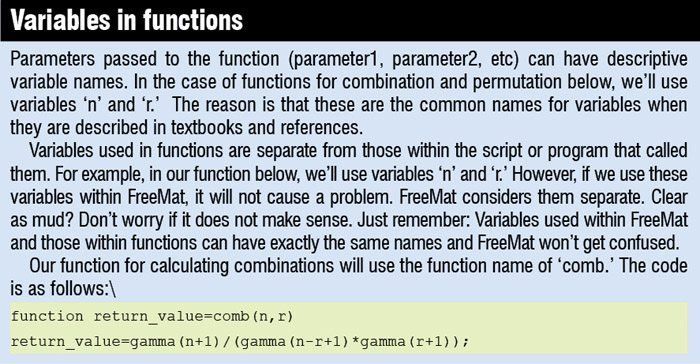## Thursday, 8 March 2018

Available under the GPL license, FreeMat is a free environment that has been designed for “rapid engineering and scientific prototyping.” It is similar to commercial tools like Matrix Laboratory (MATLAB) from Mathworks and Interactive Data Language (IDL) from Research Systems Inc.
It also features codeless interface to external C, C++ and Fortran code, parallel-distributed algorithm development (via MPI), and some extended volume and 3D visualisation capabilities.
Tutorial to make a function
Here is a short tutorial to create combination and permutation functions for FreeMat, from the FreeMat project home page at code.google.com.
In order to create functions, you can use Notepad (Windows) or vi or gedit (Linux). FreeMat also comes with a built-in editor for this purpose (see fig 1).
To open the FreeMat editor, first run FreeMat and then, from the command line, type in the word ‘edit’ (without the quotes).
In FreeMat, a function is a script that starts with word ‘function’ and uses the following syntax:
[stextbox id=”grey”]function return_value =
function(parameter1,
parameter2,…,parameterN)[/stextbox]When the editor window opens up after typing ‘edit,’ enter the following combination function:
[stextbox id=”grey”]function return_value=comb(n,r)
return_value=gamma(n+1)/(gamma
(n-r+1)*gamma(r+1));[/stextbox]
To make it a function that FreeMat can use, store the file in a folder that is part of the FreeMat path. To check the path, use the path tool. Select Tools→Path Tool and check that the directory where the function will be stored is part of the path.
To save the function, either select File→ Save or click ‘Save’ icon. When you save the file, put a ‘.m’ extension on it. This lets FreeMat know that it is a usable script.
The permutation function is similar to the combination function. And it is even simpler. The code for it is as follows:
[stextbox id=”grey”]function return_value=perm(n,r)
return_value=gamma(n+1)/gamma(n-r+1);[/stextbox]
Just as we did earlier, save the file to a directory within the working path.
Let us do a couple of simple tests on your new functions:
[stextbox id=”grey”]–> comb(7,3)
ans =
35
–> perm(7,3)
ans =
210[/stextbox]
If you get an error
The most common errors when trying to use functions is:
“Error: Undefined function or variable”This means that FreeMat cannot find the function. Double-check that you typed the function name correctly. Also check that the directory where the files are stored is in the path set by the path tool (Tools→Path Tool).
When you save the function, you need to give the file the same name as the function (except with ‘.m’ appended). The file name must match what you type to call the function exactly and is case-sensitive too; i.e., Comb(3,7) != comb(3,7). Check your naming conventions if function files are there but still things aren’t working.